### Home > CALC > Chapter 11 > Lesson 11.4.1 > Problem11-122

11-122.
1. Find the derivative,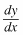, for each curve below. Homework Help ✎

1. x sin y − 10y2 = y In x

2. r = 2 − cos θ

3.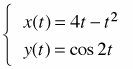4.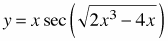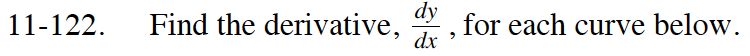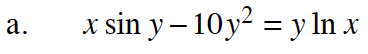$\sin(y)+x\cos(y)y^\prime-20yy^\prime=y^\prime\ln(x)+\frac{y}{x}$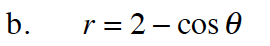$\frac{dy}{dx}=\frac{\frac{dy}{d\theta}}{\frac{dx}{d\theta}}$

$x=r\cos(\theta)=(2-\cos(\theta))\cos(\theta)$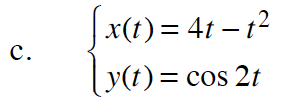$\frac{dy}{dx}=\frac{\frac{dy}{dt}}{\frac{dx}{dt}}$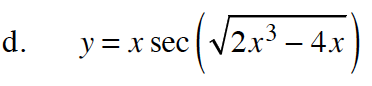$\text{Let }w=\sqrt{2x^3-4x}.$

$y^\prime=\sec(w)+x\sec(w)\tan(w)w^\prime$

What is w′ ? Substitute w and w′ into the equation in Step 2.